Courses

# Test: Chemical Equation (M)

## 20 Questions MCQ Test Chemistry Class 11 | Test: Chemical Equation (M)

Description
This mock test of Test: Chemical Equation (M) for JEE helps you for every JEE entrance exam. This contains 20 Multiple Choice Questions for JEE Test: Chemical Equation (M) (mcq) to study with solutions a complete question bank. The solved questions answers in this Test: Chemical Equation (M) quiz give you a good mix of easy questions and tough questions. JEE students definitely take this Test: Chemical Equation (M) exercise for a better result in the exam. You can find other Test: Chemical Equation (M) extra questions, long questions & short questions for JEE on EduRev as well by searching above.
QUESTION: 1

### Two flasks A and B of equal volume containing 1 mole and 2 mole of O3 respectively, are heated to the same temperature. When the reaction 2O3 →  3O2 practically stops, then both the flasks shall have

Solution:

For Reaction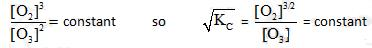= will be same for both the containers

QUESTION: 2

### A 10L container at 300K contains CO2 gas at pressure of O.2 atm and an excess solid CaO (neglect the volume of solid CaO). The volume of container is now decreased by moving the movable piston fitted in the container. What will be the maximum volume of container when pressure of CO2 attains its maximum value given that                                                CaCO3 (s)   → CaO(s) + CO2(g)             Kph = 0.800 atm

Solution:

Kp = 0.800 atm =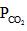= maximum pressure of CO2 in the container to calculate maximum volume of container the= 0.8 atm and none of= 0.8 should get converted into CaCO3 (s)
so V (0.800 atm) = (10 L) (o.2 atm)
so v = 2.5 L

QUESTION: 3

### An equilibrium mixture at 700 K of 0.50 M N2, 3.00 M H2 and 2.00 M NH3 is present in a container. Now if this equilibrium is disturbed by adding N2 so that its concentration becomes 1.50 M just after addition then which of the following graphs represents the above situation more appropriately –

Solution:

Let reaction be

N2+3H→ 2NH3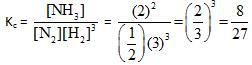Now after distrubing equilibrium then N2 concentration will be > 0.5 M and there will be some time taken for attainment of equilibrium hence graphs (B) and (C) can be discarded

from calculations it can be proved that the amount by which N2 should reaction should be less than 0.2 . Hence the result.

QUESTION: 4

At 1400 K, Kc= 2.5x10 +3 for the reaction CH4(g) + 2H2S(g) CS2(g) + 4H2(g) . A 10.0L reaction vessel at 1400 K contains 2.00 mole of CH4, 3.0 mol of CS2, 3.0 mole of H2 and 4.0 mole of H2S. Then

Solution: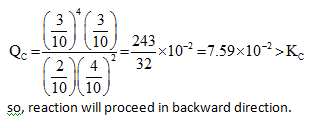QUESTION: 5

The graph which will be representing all the equilibrium concentrations for the reaction

N2O4 → 2NO2 (g) will be :

(the concentrations of N2O4 (g) and of NO (g) for which the following eaction will be at equilibrium will lie

Solution:

All the equilibrium will statisfy the equation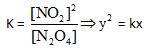the required parabolic graph

QUESTION: 6

In a basic aqueous solution chloromethane undergoes a substitution reaction in which Cl is replaced by OH as.

CH3Cl (aq) + OH- → CH3OH(aq) + Cl(aq)

The equilibrium constant of above reaction C, =1 x 10'6. If a solution is prepared by mixing equal volumes of 0.1 M CH3CI and 0.2 M NaOH (100% dissociated) then [OH-] concentration at equilibrium in mixture will be:

Solution:

CH3Cl (aq) + OH- → CH3OH(aq) + Cl-(aq)

As equilibrium constant is very large so reaction will be almost 100% complete.

QUESTION: 7

For the chemical equilibrium,
CaCO3 (S) → CaO(s) + CO2 (g)

ΔHf° can be determined from which one of the following plots ?

Solution:

CaCO3 (S) → CaO(s) + CO2 (g)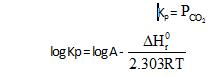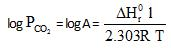Graph (a) represents (i) and its slope will be used to determine the heat of the reaction.

QUESTION: 8

At a certain temperature the following equilibrium is established, CO(g) + N02(g) →  CO(g) + NO(g) One mole of each of the four gas is mixed in one litre container and the reaction is allowed to reach equilibrium state. When excess of baryta water (Ba(OH)2) is added to the equilibrium mixture, the weight of white ppt (BaCO3) obtained is 236.4 gm. The equilibrium constant Cc of the reaction is (Ba = 137)

Solution:

CO(g) + N02(g) →  CO(g) + NO(g)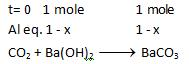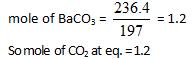or     1+x = 1.2
x= 0.2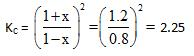QUESTION: 9

At temperature T, the compound AB2 (g) dissociates according to the reaction, 2AB2 (g)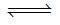2 AB(g) + B2(g).With a degree of dissociation x, which is small compared with unity. Deduce the expression for x in terms of the equilibrium constant, Kp and the total pressure, P.

Solution: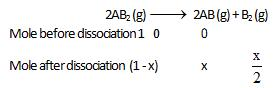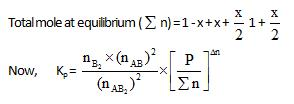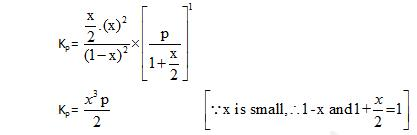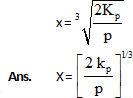QUESTION: 10

Equilibrium constant for the given reaction is C = 1020 at temperature 300 K

A(s) +2B(aq)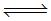2C (s) + D(aq.)K = 1020

The equilibrium conc. of B starting with mixture of 1 mole of A and 1/3   mote/titre of B at 300 K is

Solution: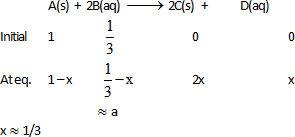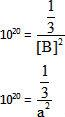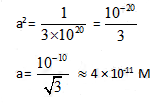QUESTION: 11

10lt box contain O3 and O2 at equilibrium at 2000 K. The ΔG0 = –534.52  kJ at 8 atm equilibrium

pressure.The following equilibrium is present in the container

2o3(g)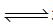3O2 (g). the partial pressure of O3 will be ( In 10 = 2.3, R = 8.3  J mol-1 K-1)

Solution:

ΔG0 = -Rt In K = -2.3 x 2000 x 8.3 log K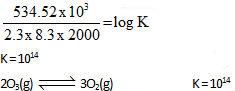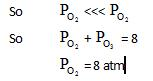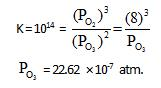QUESTION: 12

Solid ammonium carbamate dissociates to give ammonia and carbon dioxid as follows:

NH2COONH4(s)2NH5(g) + CO2(g)

At equilibrium, ammonia is added such that partial pressures of NH5 now equals the original total pressure. Calculate the ratio of the total pressures now to the original total pressure.

Solution: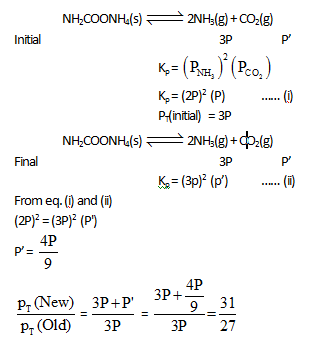QUESTION: 13

The reactions,   PCl5 (g)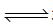PCl5(g) + Cl2(g) and Cl2(g)CO2(g) + Cl2 (g) are simultaneously in equilibrium in an equilibrium box at constant volume. Afew  moles of   CO(g) are later introduced into the vessel. After some time, the new  equilibrium concentration of

Solution:

If CO is added 2nd equilibrium will proceede in the backward dkection and concentration of Cl2 will decrease. This Cl2 will be furtherformed by the decomposition of PCI5

QUESTION: 14

In the Haber process for the industrial manufacture of ammonia involving the  reaction,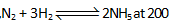atm pressure in the presence of a catalyst a temperature of about 500°C is used. This is considered as optimum temperature for the process because

Solution:

Formation of ammonia is an exothermix process therefore it is favorable at lower temperature. But at lower temperature rate of the reaction becomes slow.

QUESTION: 15

For the equilibrium of the reaction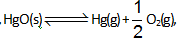,kP for the reaction at total pressure of P is:

Solution: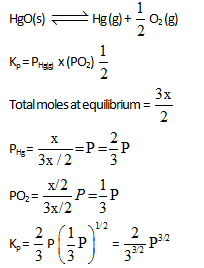QUESTION: 16

What is the minimum mass of CaC05 (s), below which it decomposes completely, required to establish equilibrium in a 6.50 litre container for the reaction : [ KC = mole/litre]

CaCO3 (s)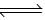CaO(s) + CO2(g)

Solution:

Kc = [Cl2] = 0.05 mole/itre
so moles of CO2= 6.50 x 0.05 moles = 0.3250 moles

CaCl3CaO + Cl2

1 mole of CO2 =1 mole of CaCO3

0.3250 moles of CO2 = 0.3250 moles of CaCO3= 0.3250 x 100 gm of CaCO3 = 32.5 gm of CaCO

QUESTION: 17

The value of kp for the reaction at 27°C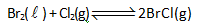is '1 atom'. At equilibrium in a closed container partial pressure of BrCI gas is 0.1 atm and at this temperature the vapour pressure of Br2(l) is also 0.1 atm. Then what will be minimum moles of Br2(l) to be added to 1 mole of C12 , initially, to get above equilibrium situation :

Solution: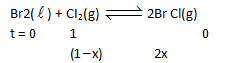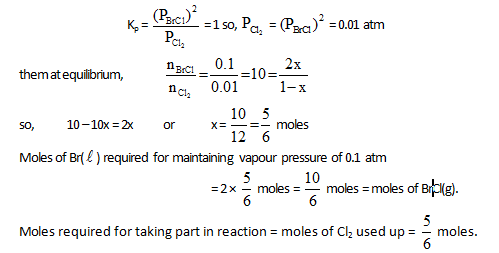QUESTION: 18

5 mol PCI3(g) and one mole N2 gas is placed in a closed vessel. At equilibrium PCI5(g) decomposes 20% and total pressure in to the container is found to be 1 atm. The kP for equilibrium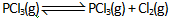Solution: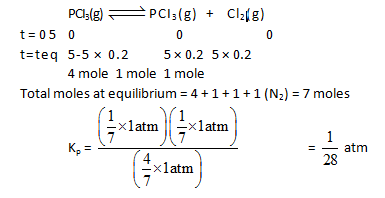QUESTION: 19

Degree of association can be defined as the number of moles of a particular substance associated per mole of the substance taken.

For example : If out of 10 mole of N2 , 3 mol of N2 combine with H2 to form NHL. then degree of association of N2 = 0.3.Consider the equilibrium situation :  N2(g) + 3H2(g)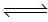2NH3(g)

Initially N2 & H2 were mixed in 1 : 3 molar ratio and after long time the mean molar mass of the mixture was  found to be 34/3g. The degree of association of N2 is

Solution:

The correct answer is Option A
N2​(g) + 2H2​(g) ⇌ 2NH3​(g)
t=0        1             3              0
t=teq​  1 - x       3 - 3x         2x
Total moles at equilibrium = 4 − 2x
Mean molar mass  =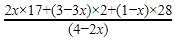Value of mean molar mass is given. Find ′x′.

QUESTION: 20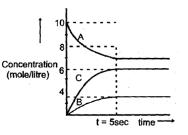Attainment of the equilibrium A(g)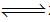2C(g) +B(g) gave the gave the followeing graph. Find the correct option. (% dissociation = fraction dissociated x 100)

Solution:

The correct answer is Option D.

Kc = ([C]2 * [B]) / [A]
= (62 * 4) / 8
= 18 M2

And, % dissociation of A = ([10 - 8] / 10)*100
=  20 %